# Input-Output Model

## Definition

An Input-Output Model (also Input-Output Table) is a model constructed from observed economic data which provides information about the activity of industries that produce and consume goods and services. The core component of such models. the Input-Output Matrix (or Table) is a means of presenting a detailed analysis of the process of production and the use of goods and services (products) and the income generated in that production.

Such tables can be either in the form of

• supply and use tables or
• symmetric input-output tables.

Traditional input-output (IO) tables summarise the exchanges between major sectors of an economy.

An IO can be seen as either:

• a stastical summary (of some aspects) of an economy or (with additional assumptions) or
• an actual model of how supply and demand and production and consumption are related.

In the first instance the term table might be more appropriate, in the second usage the term model highlights this more specific usage (subject to Model Risk)

## Structure

The basic IO table, including the table of final demand, the value added and the input coefficients matrix, is usually provided by national statistical offices for the national economy. Input-Output accounts are usually constructed using annual periods but this is conventional, in princinple any period over which data can be collected is allowed. Interindustry relationships are derived from interindustry transaction tables in which the rows describe the composition of inputs required by a particular industry to produce its output. The data often concern industries in a specific geographic region (nation, state, county, etc.) and consist in monetary flows (but see also Physical Input-Output Tables.

• Additional matrix (table) columns represent final demand, i.e. the sales to final markets (consumers, government, exports).
• Additional matrix (table) rows represent non-industrial production inputs (labor, capital, etc.)1.

## Formula

An Input-Output model is fundamentally a system of linear equations where the Total Output of an industry is distributed through sales to other sectors and to final demand: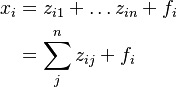\begin{align} x_{i} & = z_{i1} + \ldots z_{in} + f_i \\ & = \sum_{j}^{n} z_{ij} + f_i \end{align}

where

•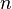$n$ is the number of industries in the modeled economy
•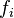$f_{i}$ is the final demand of sector's i production
•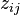$z_{ij}$ is the flow of primary input i to sector j (value added bloc of the IO table), the demand of sector j for inputs from sector i
•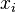$x_i$ is the monetary value of the output of sector j (production value) during a period (year)

This system of equations expresses a form of market balance.

## Usage

• Input-output analysis can serve various analytical purposes such as impact analysis, productivity analysis, employment effects, analysis of the interdependence of structures and analysis of price change.
• In a corporate context an IO model can be used for analysing the effects of a company's activities on upstream production (supply) industries along the value chain.
• Intermediate goods incorporated in the production process can be allocated to different sectors of the economy (the supply links among the individual sectors can be shown)
• On the basis of the structure of the goods and services actually required or obtained, the intermediate goods and services (tier 2 – tier n) statistically required for the production of the company’s production input (tier 1) can be calculated using IO analysis. As a result, the (statistically) required total input of goods and services is determined along the upstream stages of the value chain.
• IO tables and models are widely used in the context of classical economic impact analysis (EIA) and in environmentally extended IO analyses (EEIO). Input-output analysis can be used to allocate upstream emissions or downstream emissions systematically while avoiding the double-counting problem. In doing so, ultimate upstream responsibilities are allocated to the consumers of the final products (such as households) by using the Leontief inverse and ultimate downstream responsibilities are allocated to the providers of primary factors (such as workers, investors, land owners, etc.) by using the Ghosh inverse

## Issues and Challenges

• Aggregation Bias
• Single-region models focus on only the production and trade within the borders of the country in question, multi-regional models are required to take into account the imports of commodities from outside the national boundaries. This raises data requirements and the potential of inconsistent accounting.
• Monetary focus: Leontief’s original input–output framework (Leontief, 1936) conceived of industry production functions, which he frequently referred to as production “recipes,” as mea- sured in physical units, such as specifying the technical coefficients in tons of coal or bushels of wheat, as inputs, required per dollar’s worth of an industry’s output or per ton of steel output. However, the data collection requirements and a number of other constraints rendered implementation of the framework in physical units too unwieldy, certainly at the time and even today to a lesser extent. Hence, the basic methodology for input–output analysis evolved, in both theory and application, through measuring all quantities in value terms with implicit fixed prices, as detailed in earlier chapters.
• the conceptually simplifying assumptions of fixed input requirements and constant relative prices, both implicit in the classical input–output framework, render implementation easier, although satisfying the data requirements can still be quite difficult. On the other hand, these assumptions limit the ability of the framework to deal very effectively with some fundamental features of a modern economic system, such as prices or elasticities.